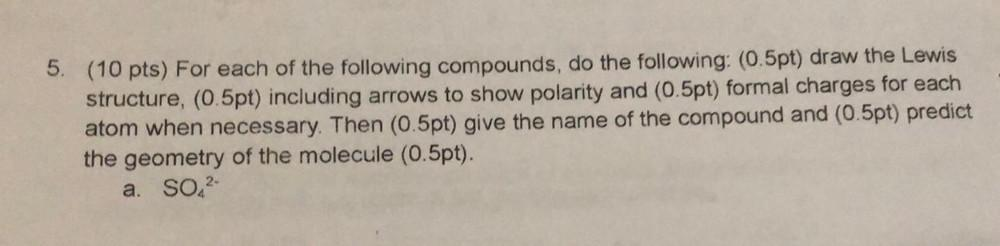Question:

# 5. (10 pts) For each of the following compounds, do the following: (0.5pt) draw the Lewis structure, (0.5pt) including arrows to5. (10 pts) For each of the following compounds, do the following: (0.5pt) draw the Lewis structure, (0.5pt) including arrows to show polarity and (0.5pt) formal charges for each atom when necessary. Then (0.5pt) give the name of the compound and (0.5pt) predict the geometry of the molecule (0.5pt). a. SO2-